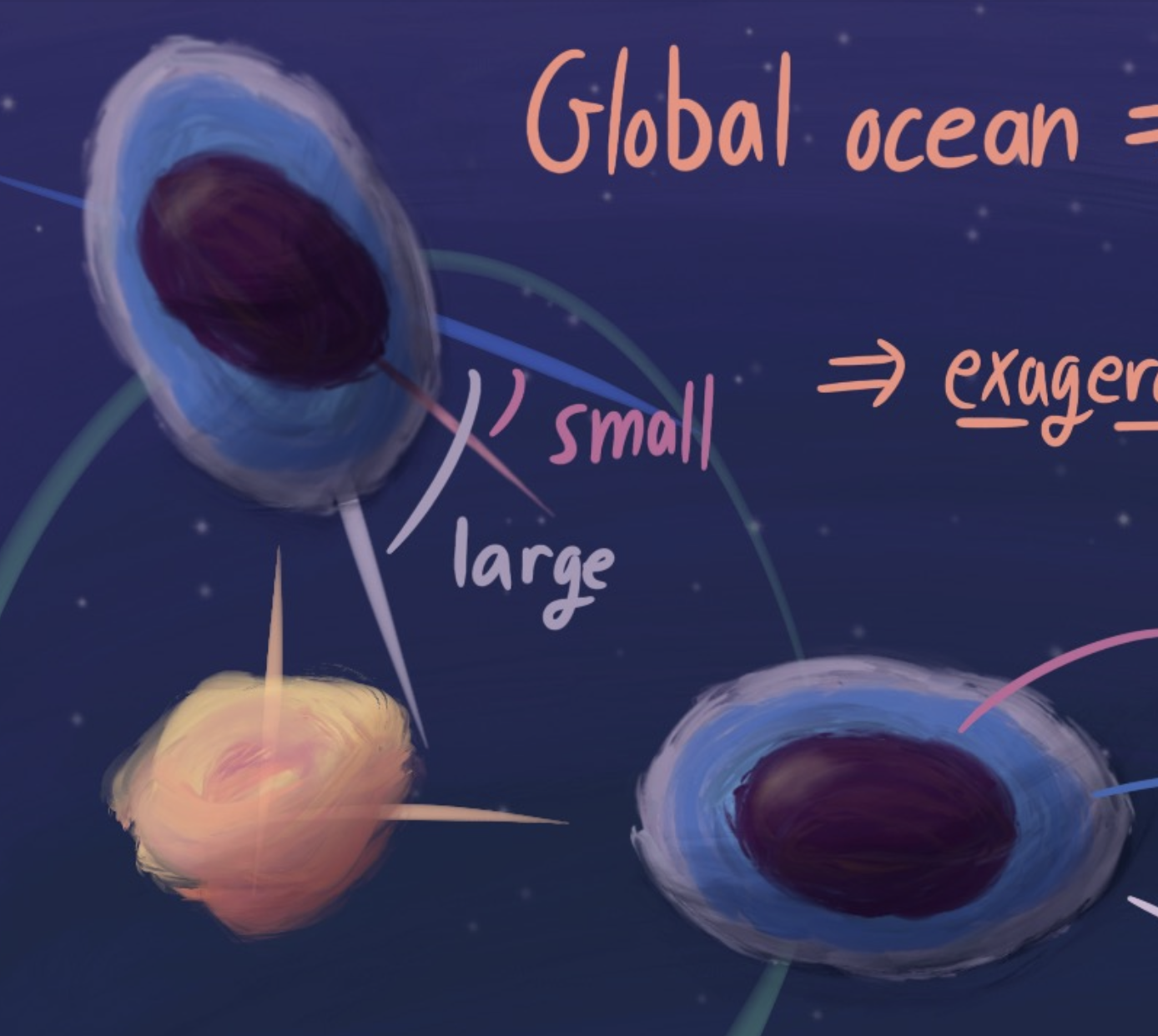##### Hyperbolic band theory through Higgs bundles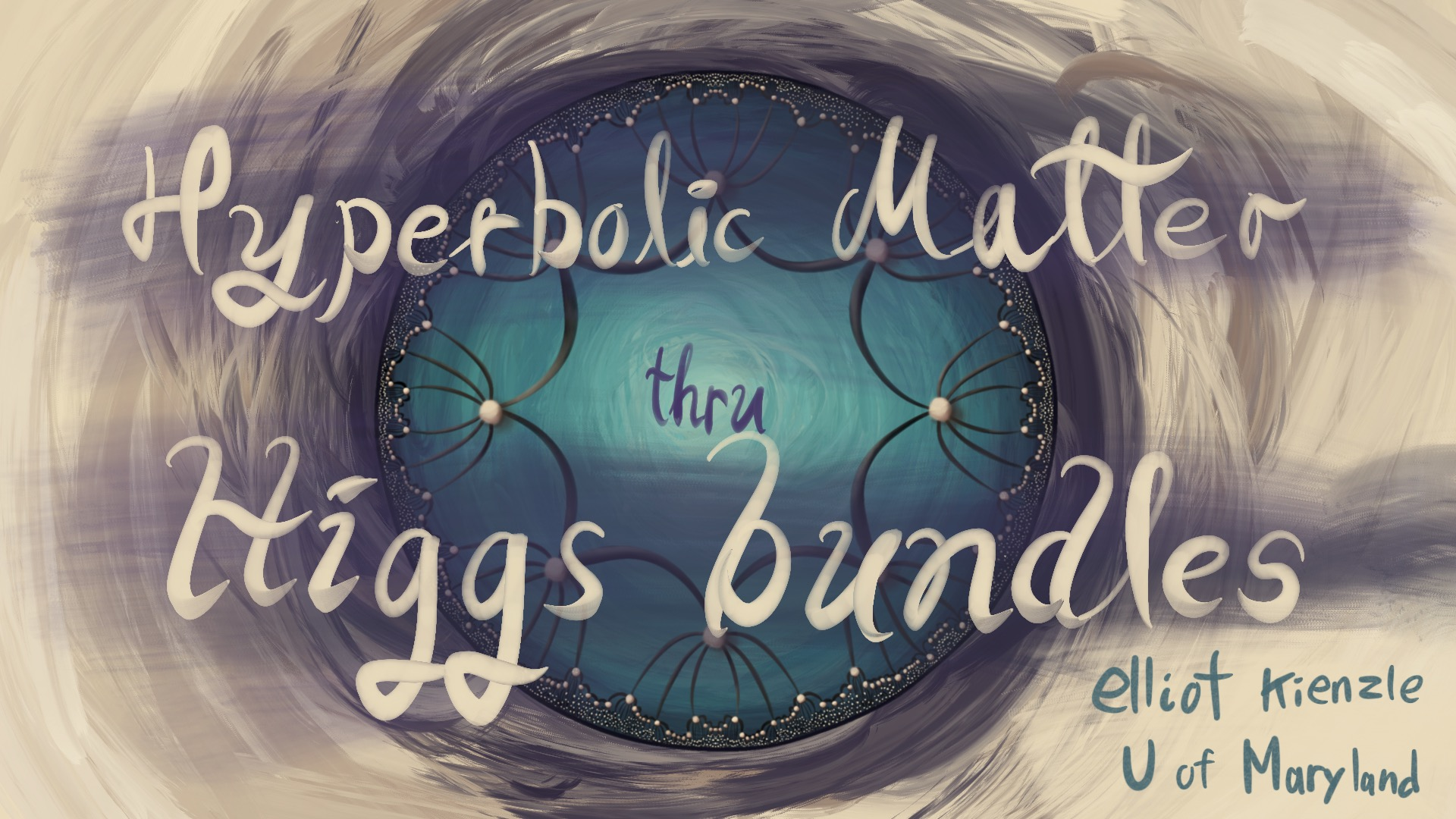r
##### The Dark Magic of Integrable Systems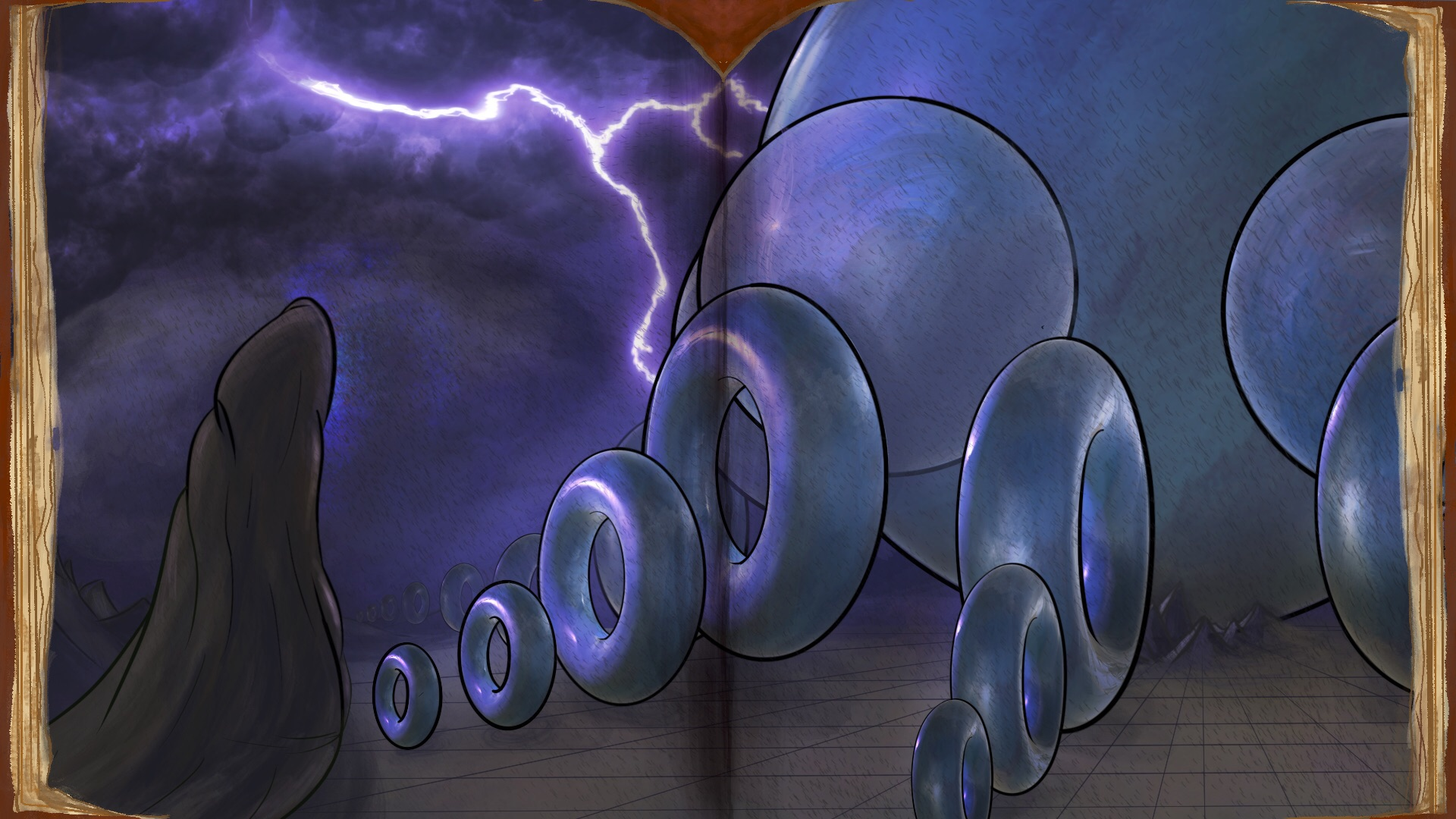The nefarious physicsts, always a cult of symmetry, have gone too far this time. By constructing a theory with not 1, but 2 supersymmetric partners, they evoked the dark magic of integrable systems. Now they must clean up their mess. They leave gauge theory grove for Loui-ville, a humdrum town caught in the eternal cycles around the tori in an integrable system. Next they brave the soliton swamps, coming face to face with the Toad-a lattices and their waves of hopping toads. They escape the swamp through the spectral cemetary, where the spirits of long-passed integrable systems are chained to forever cover their riemann surfaces. At last, they come upon the Seiberg-Witten summit, a fortress of spectral curves built by the supersymmetric theory they brought into the world.
##### BPS states, Seiberg-Witten theory, and Integrable systems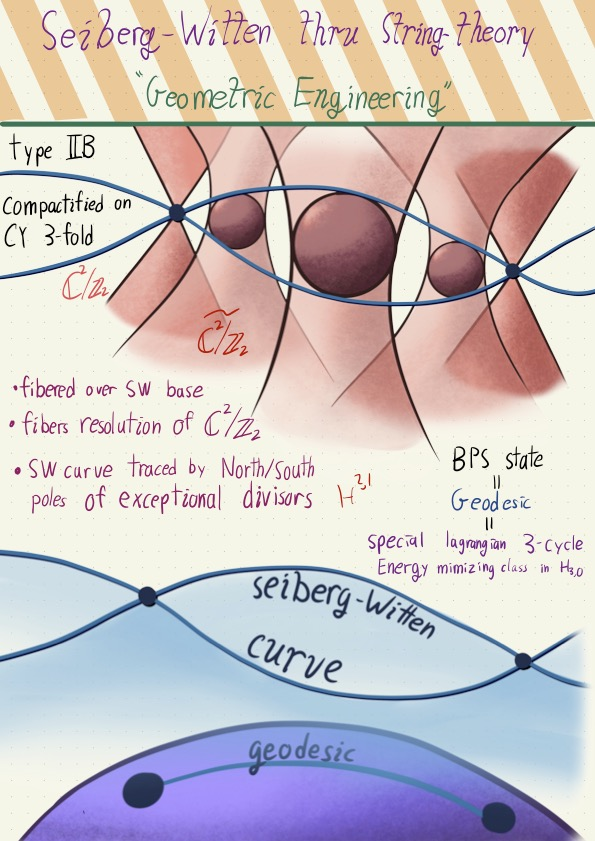a trilogy of talks that all explore the power of BPS states in math and physics. First, I relate the BPS representations of supersymmetric algebras with BPS monopoles. Second, I go through Seiberg and Wittens solution for the low energy effective field theory of N=2, D=4 super yang mills. Vitally, they use the topological aspect of BPS states to pull back a lattice from the free theory to the whole vacuum moduli space, giving an integrable system. Third, I give a unified description of BPS states as variations of hodge structures, and discuss how various avatars of BPS states fit in to the story.
##### Topological recursion: For fun and profitHow do rainbows relate to quantum gravity, random matricies, and WKB analysis? Im glad you asked. These all have underlying recursive formula, based on cutting up riemann surfaces into pairs of pants. These various types of 'topological recursion' were unified by Eynard and Orantin, relating each recursive structure to a spectral curve of a simple polynomial.
##### A and B models: The story of mirror symmetry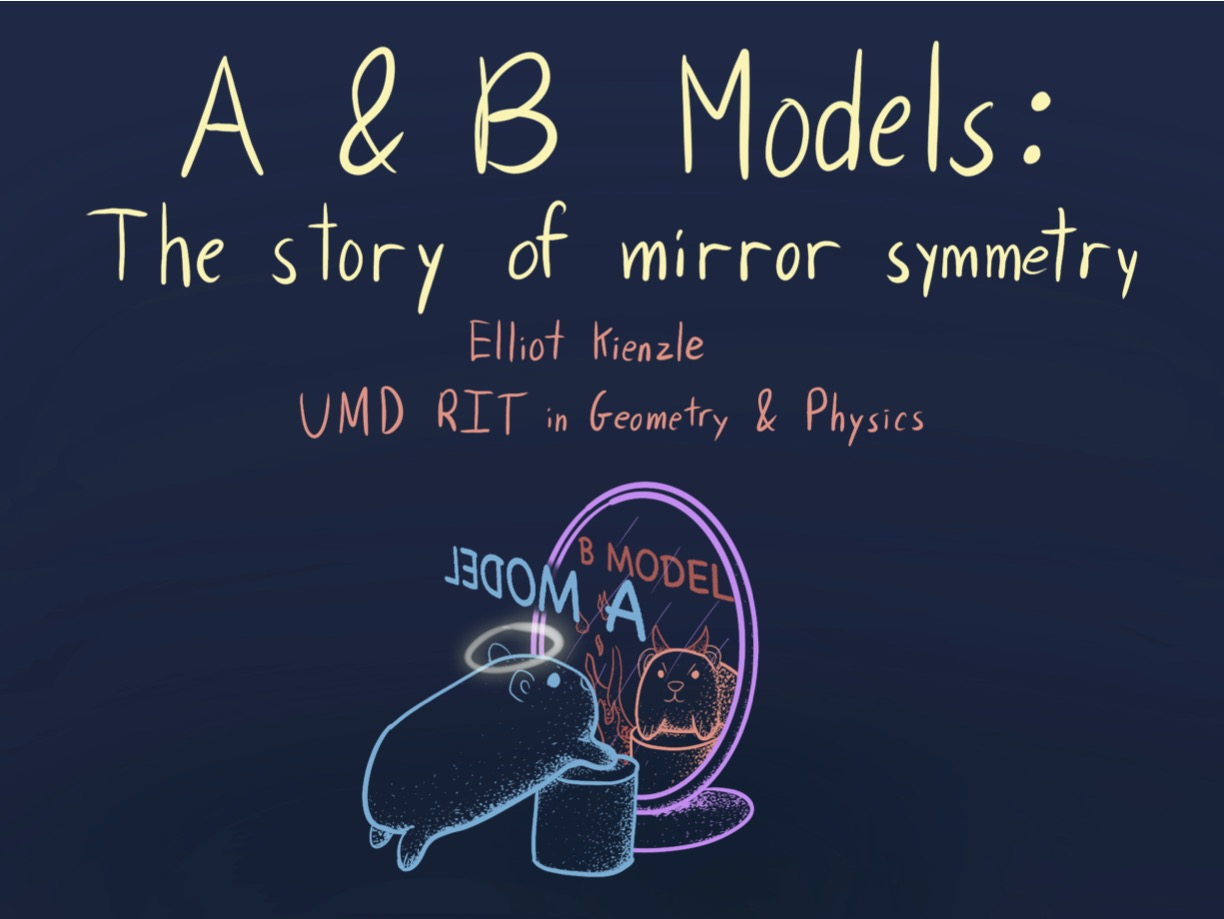A statement of mirror symmetry, thought of as an equivalence of frobenius manifolds. Two sorts of 'topological twisting' should give equivlent topological field theories. The first gives the A-model, which is a path integral counting holomorphic curves in a kahler manifold. The second gives the B-model, a Landau-Ginzburg theory whose physics depends on the singularity structure of a holomorphic function. Underlying both is the structure of a frobenius manifold-- Mirror symmetry conjectures that its the same structure
##### Cobordisms and the Thom SpectrumIn the first part, I give a whimsical introduction to cobordisms, what they do for algebraic topologists, and how they come up in mathematics. In the second, I construct the Thom spectrum associated to the cobordism cohomology theory, using the Pontryagin-Thom construction.
##### Higgs bundles, Mirror symmetry, and Geometric Langlands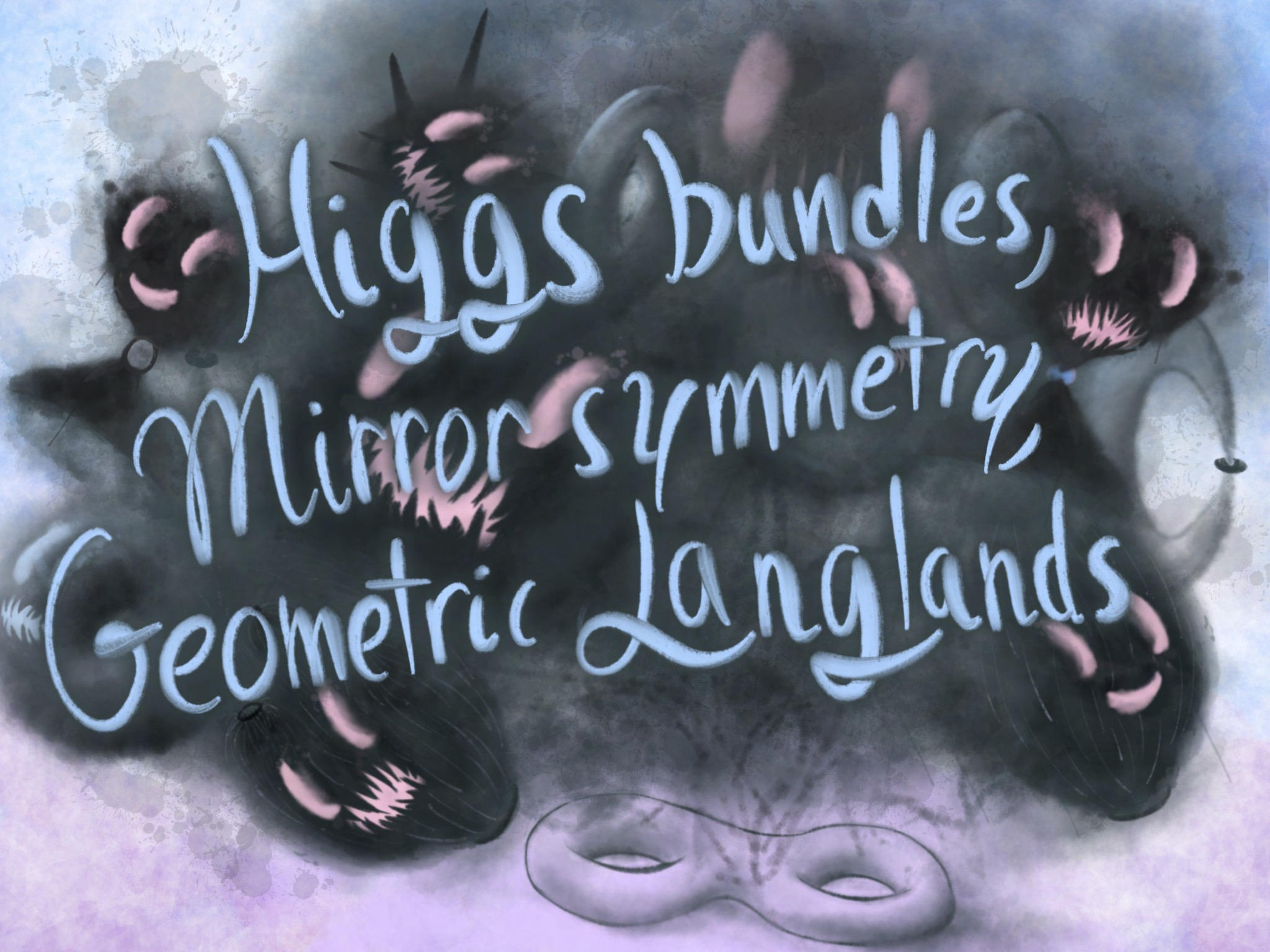A summary of the Kapustin-Witten approach to the geometric Langlands program.
##### Electromagnetism on a Riemann surface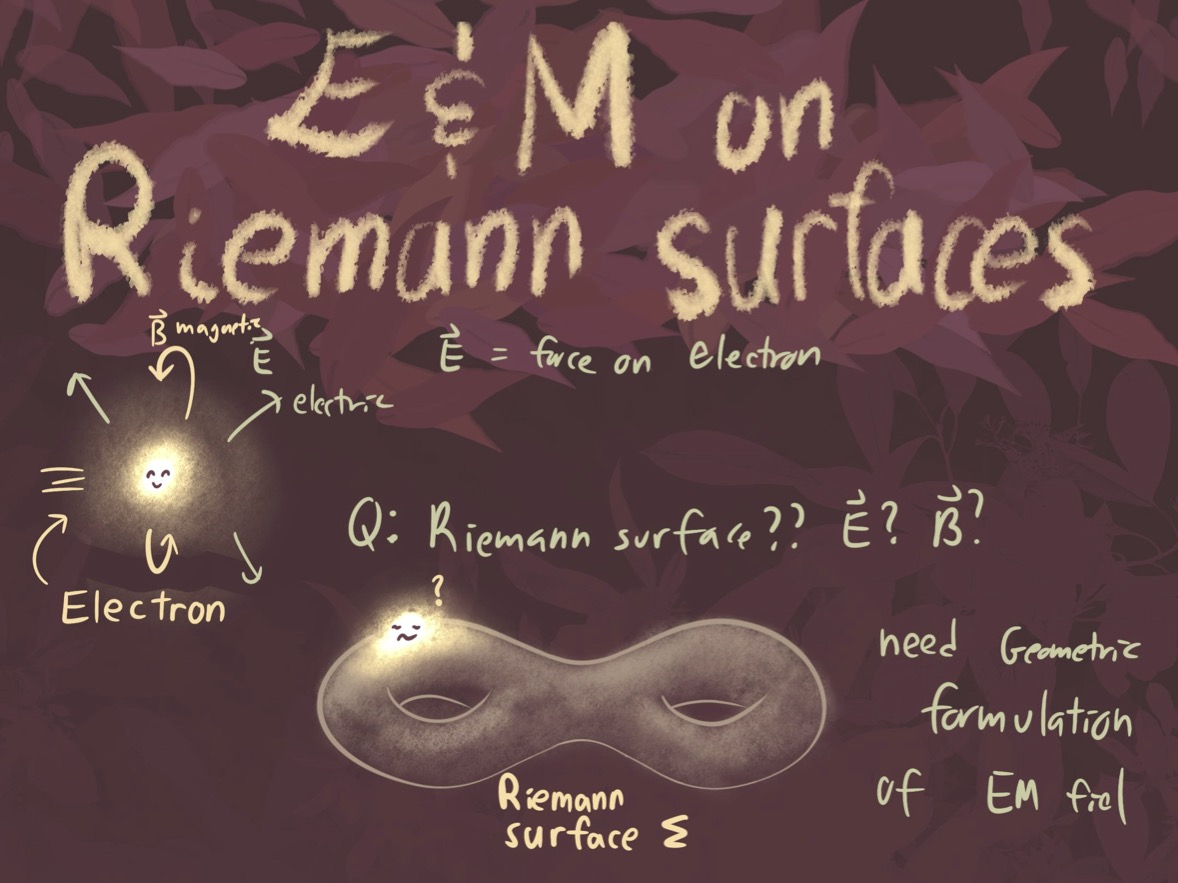Maxwells equations have a very elegant formulation in terms of differential forms. As a U(1) gauge theory, the electromagnetic field is a connection on a U(1) principle bundle. These are equivalent to holomorphic line bundles! The moduli space of solutions to vacuum maxell's equations on a riemann surface is the Jacobian. We can give much of classical Riemann surface theory a gauge-theoretic coat of paint. This observation, generalized to nonableian groups and noncompact groups, is the heart of the nonabelain hodge correspondence.
##### The Riemann-Roch Theorem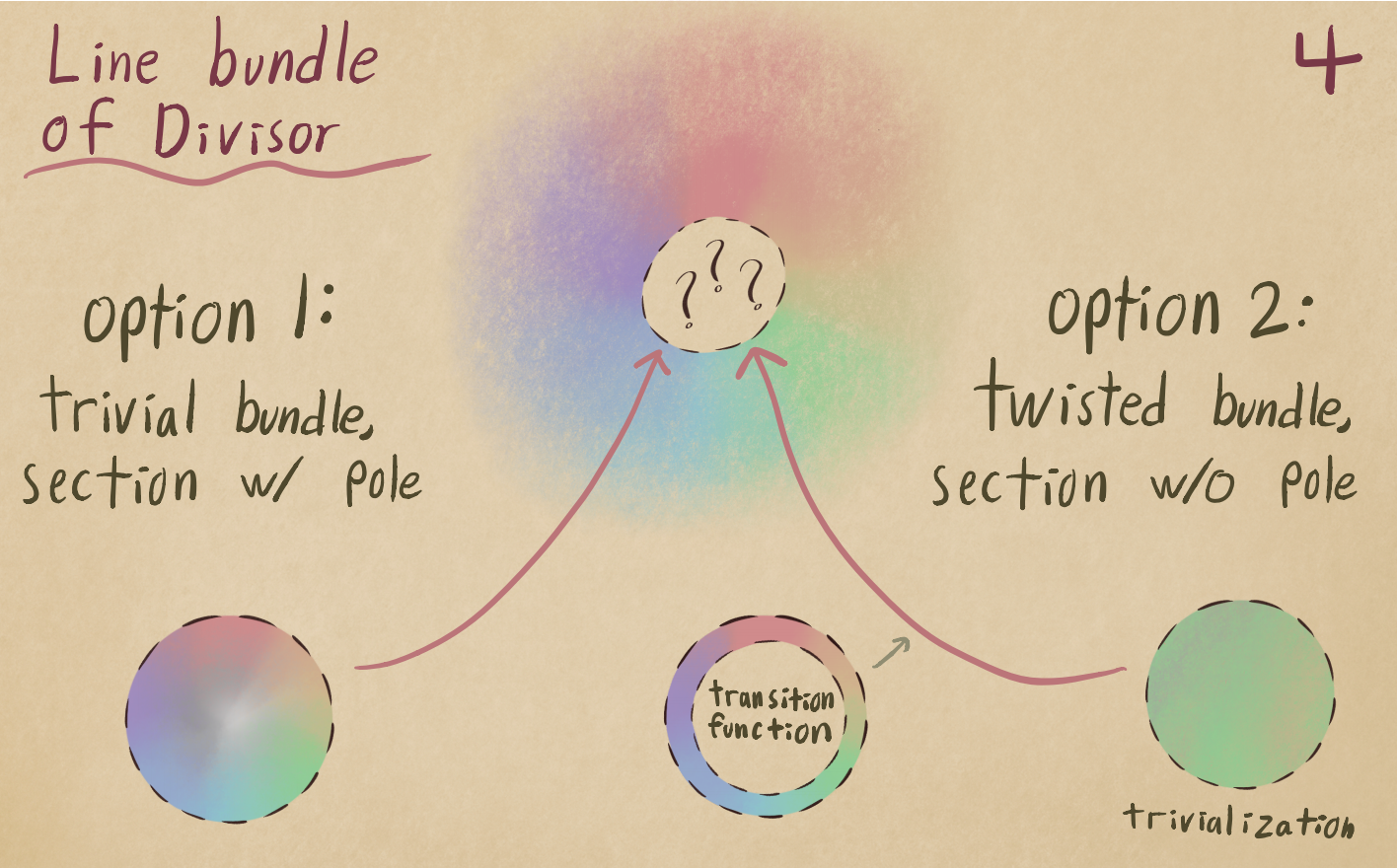The Riemann-Roch theorem gives the number of linearly independent holomorphic sections to a line bundle. I explain morally why divisors should correspond to line bundles, and present the usual sheaf-theoretic derivation of Riemann-Roch. But to me, Riemann-Roch relates the solutions of differential equations to the genus, bridging analysis and toppology. This was my first intoxicating taste of the Atiyah-Singer index theorem.
##### Lie's Theorem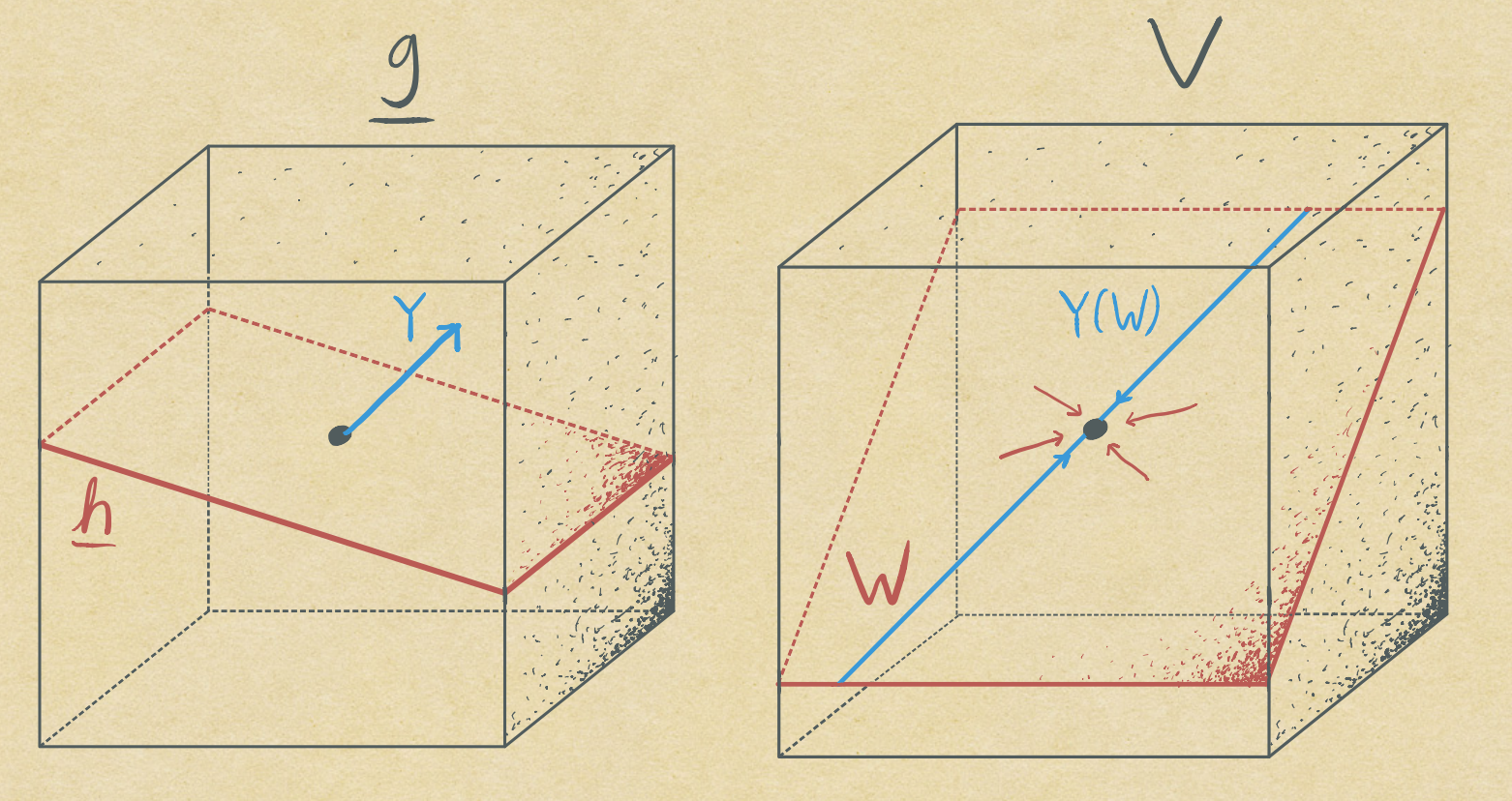A short (~15 minute) exposition & proof of Lie's theorem, which says that solvable Lie algebras act like upper triangular matricies.
##### Kahler Quantization and Physics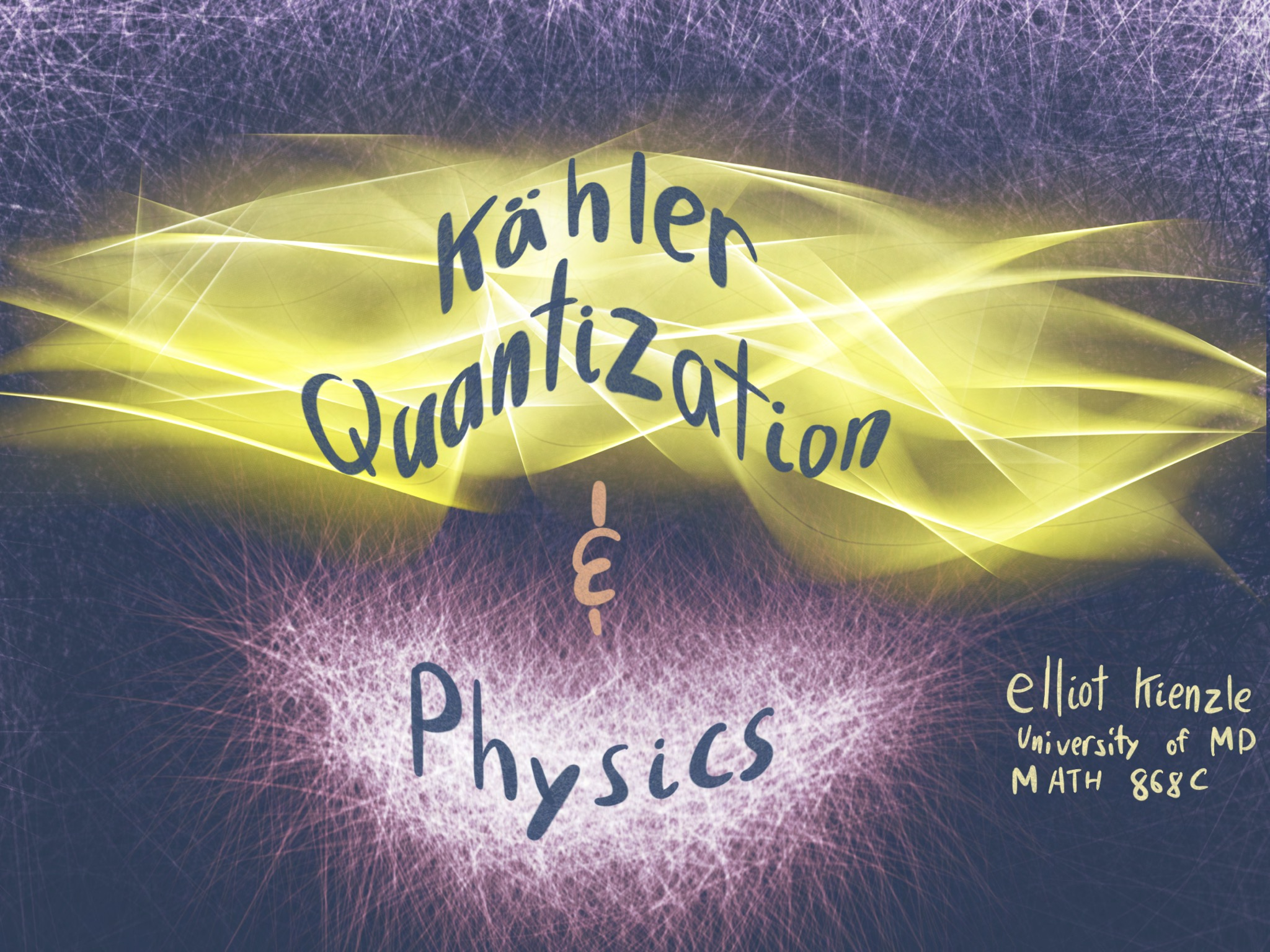Geometric quantization associates a Kahler manifold to a vector space of holomorphic sections of a line bundle. By taking higher and higher powers of the line bundle, there are more and more holomorphic sections. In the limit, each point on the manifold sits in CP infinity by the Kodira embedding. These are coherent states, acting as quantum analogous of a classical point particle. This strategy is very useful in pure Kahler geometry.
##### Higgs bundles and geometric structures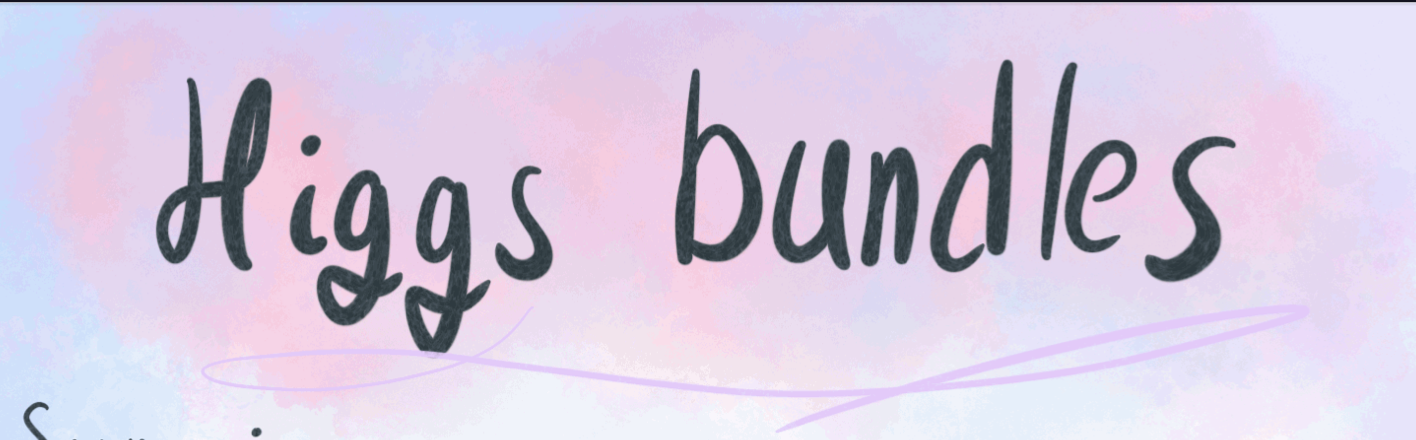An introduction to the theory of Higgs bundles and the nonabelian hodge correspondence. I emphasized the aspects related to geometric structures, in particular how the Hitchin section of SL(2,C) Higgs bundles gives hyperbolic structures.